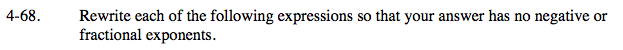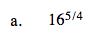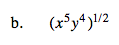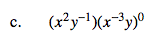### Home > A2C > Chapter Ch4 > Lesson 4.2.1 > Problem4-68

4-68.Rewrite the expression:

$(16^{\frac{1}{4}})^5=(\sqrt{16})^5$

First find the fourth root of 16.

$\sqrt{16}=2$

Now raise the 2 to the 5th power.

25 = 32

32$\text{Remember that a number raised to the }\frac{1}{2}\text{ power}$

$\text{is the square root of that number.}$

$\textit{x}^{2}\textit{y}^{2}\sqrt{\textit{x}}$Remember that any number to the 0 power is 1. Remember to rewrite it without negative exponents.

(x2y−1)(1)